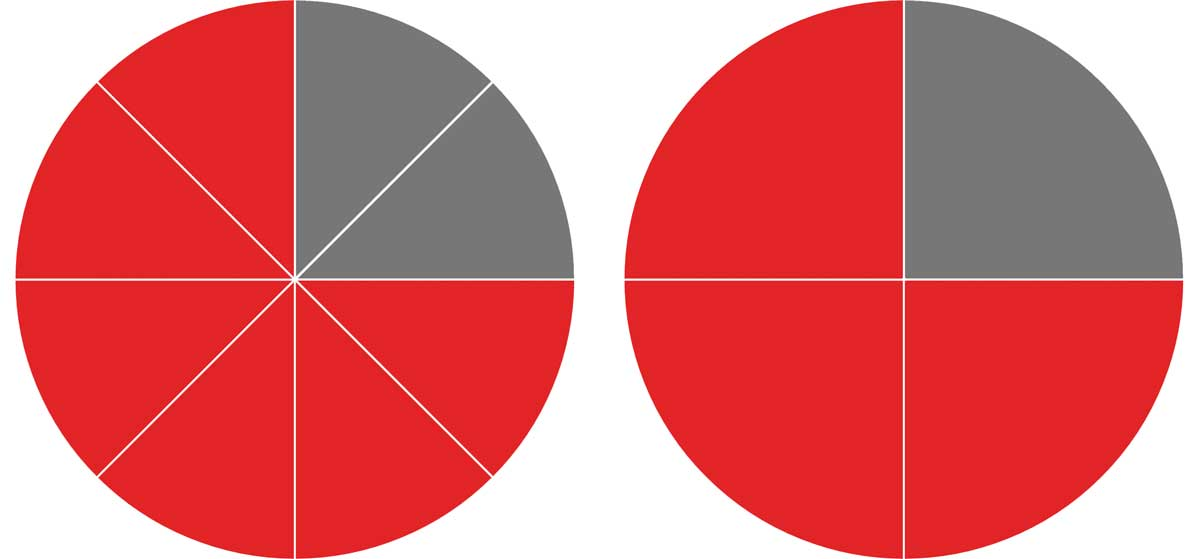# Reduce Fractions Calculator

Enter a fraction below to simplify or reduce a fraction into the simplest form. The calculator shows all of the steps used to simplify the fraction.

Fraction:

## Solution:

253=813

Reduce the fraction by finding the greatest common factor. The greatest common factor of 25 and 3 is 1
Divide the numerator and denominator by the greatest common factor (1)
25 ÷ 13 ÷ 1=253
Convert the fraction to a mixed fraction by finding the whole number and remainder
25÷3=8R1
253=813
Find the decimal by dividing the numerator by the denominator
253=25÷3=8.3333333333333

## How to Reduce a FractionSome fractions can be reduced to a simpler form, which means to simplify the fraction to the lowest possible terms. A simplified or reduced fraction will not have any common factors between the numerator or denominator other than 1.

For example, the fractions 13 and 26 are equivalent fractions, meaning they are equal. However, 13 is in the lowest possible terms because the only common factor of 1 and 3 is 1.

Follow a few simple steps to reduce a fraction.

### Step One: Find the Greatest Common Factor

The first step to reducing a fraction is to find the greatest common factor between the numerator and the denominator. The greatest common factor will be used in the second step. The greatest common factor is the largest number that is evenly divisible by both the numerator and denominator.

For example, for the fraction is 68, let’s find the factors of 6 and of 8.

The factors of 6 are [1,2,3,6].

The factors of 8 are [1,2,4,8].

The largest number that is common to both is 2, thus 2 is the greatest common factor.

See our greatest common factor calculator for additional information on finding the greatest common factor.

### Step Two: Divide by the Greatest Common Factor

Armed with the greatest common factor it’s now possible to reduce the fraction. To do so, divide both the numerator and the denominator by the greatest common factor. The result is a reduced fraction.

Continuing the above example, simplify the fraction 68 by dividing the numerator and denominator by the greatest common factor of 2 that was found in the previous example.

68 = (6 ÷ 2)(8 ÷ 2)
68 = 34

Thus, 34 is the simplified form of 68.

### Handling Fractions Larger Than 1

Some fractions are larger than 1, which is when the numerator is larger than the denominator. When the numerator is larger than the denominator it is often helpful to reduce the fraction further into a mixed number. To do this, divide the denominator by the numerator and set the quotient as the whole number and the remainder as the numerator over the original denominator.

For example, 128 can be simplified to 32. Let’s convert this to a mixed number.

32 = 3 ÷ 2 = 1 R1
32 = 1 12

Here’s a tip: our fraction to mixed fraction calculator can help convert an improper fraction like this to a mixed number.